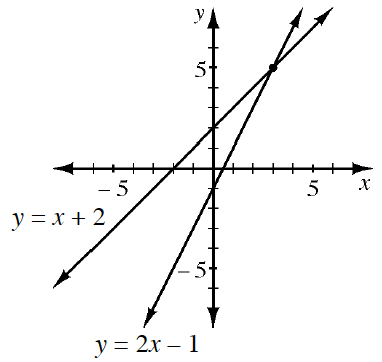### Home > CCA > Chapter 4 > Lesson 4.2.2 > Problem4-50

4-50.

The graph at right contains the lines for $y=x+2$ and $y=2x−1$.

1. Using the graph, what is the solution to this system?

What is the point at which the two lines intersect?

$(3,5)$

2. Solve the system algebraically to confirm your answer to part (a).

Use the Equal Values method by setting the equations equal to each other.

Did you get the same solutions for $x$ and $y$ as in part (a)?